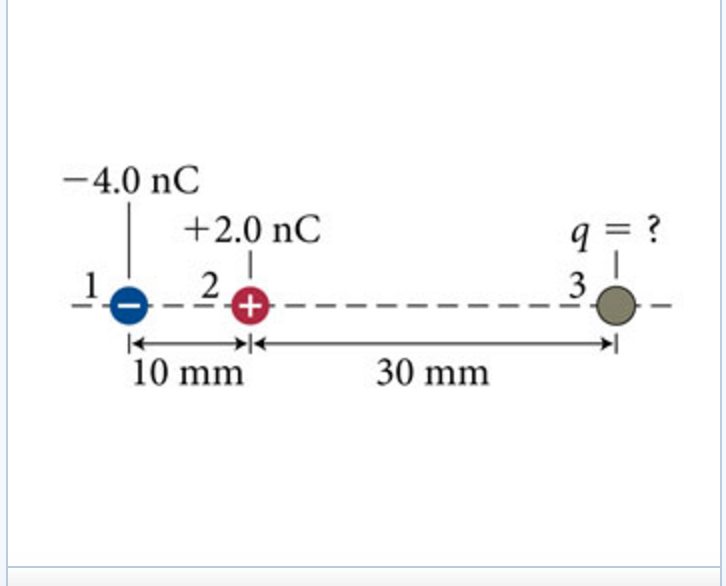# Problem: Three charged particles are arranged along a line as in this figure.What is the charge on particle 3?

###### FREE Expert Solution

In this problem, we're going to use Coulomb's law:

$\overline{){\mathbf{F}}{\mathbf{=}}\frac{\mathbf{k}{\mathbf{q}}_{\mathbf{1}}{\mathbf{q}}_{\mathbf{2}}}{{\mathbf{r}}^{\mathbf{2}}}}$, where F is the electric force, k is Coulomb constant, and q1 and q2 are charges separated by distance r.

From Coulomb's law, F12 is:

$\begin{array}{rcl}{\mathbf{F}}_{\mathbf{12}}& \mathbf{=}& \frac{\mathbf{k}{\mathbf{q}}_{\mathbf{1}}{\mathbf{q}}_{\mathbf{2}}}{{{\mathbf{r}}_{\mathbf{12}}}^{\mathbf{2}}}\\ & \mathbf{=}& \frac{\mathbf{\left(}\mathbf{9}\mathbf{×}{\mathbf{10}}^{\mathbf{9}}\mathbf{\right)}\mathbf{\left(}\mathbf{4}\mathbf{.}\mathbf{0}\mathbf{×}{\mathbf{10}}^{\mathbf{-}\mathbf{9}}\mathbf{\right)}\mathbf{\left(}\mathbf{2}\mathbf{.}\mathbf{0}\mathbf{×}{\mathbf{10}}^{\mathbf{-}\mathbf{9}}\mathbf{\right)}}{{\mathbf{\left(}\mathbf{10}\mathbf{×}{\mathbf{10}}^{\mathbf{-}\mathbf{3}}\mathbf{\right)}}^{\mathbf{2}}}\end{array}$

88% (342 ratings)###### Problem Details

Three charged particles are arranged along a line as in this figure.

What is the charge on particle 3?Frequently Asked Questions

What scientific concept do you need to know in order to solve this problem?

Our tutors have indicated that to solve this problem you will need to apply the Coulomb's Law (Electric Force) concept. You can view video lessons to learn Coulomb's Law (Electric Force). Or if you need more Coulomb's Law (Electric Force) practice, you can also practice Coulomb's Law (Electric Force) practice problems.